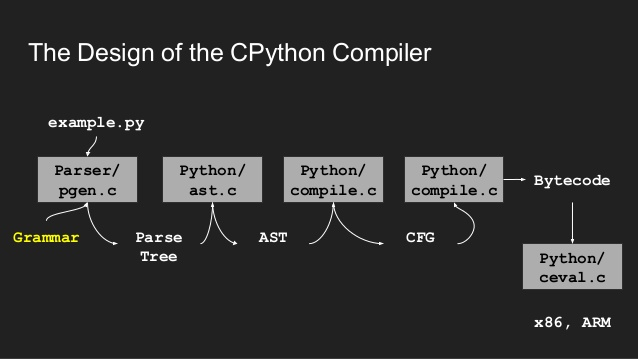# Python AST Parsing

How I used the Python AST capabilities to build the Python to Rpn converter.

## Python compilation pipeline

My program works at the AST stage of the compilation pipeline:Specifically my approach has been to use Python’s built in ability to parse itself into an AST Abstract Syntax Tree, then to traverse this tree using the visitor design pattern to generate the RPN.

The Python AST parser is built into Python and thus requires that you be running Python to use it.

## Example

For example, parsing the following Python code with `ast.parse`:

``````import ast
import astunparse
print(astunparse.dump(ast.parse('x = 1 + 2')))
``````

will generate the following AST data structure representing `x = 1 + 2`

``````Module(body=[Assign(
targets=[Name(
id='x',
ctx=Store())],
value=BinOp(
left=Num(n=1),
right=Num(n=2)))])
``````

## Reception to the talk

The reception to the talk entitled “Leveraging the Python AST, Python’s hidden DOM” was good, but became fun and buoyant once the audience realised I had targeted an old HP calculator !with this fancy Python technology - especially when I pulled out my HP calculator collection for all to see and touch. 😄

-Andy Bulka

## Resources

• Python AST talk slides of the talk I gave to a local Python User Group in Melbourne, Australia, about how I used the Python AST capabilities to build the Python to Rpn converter.
• Python to Rpn converter which uses these AST conversion capabilities to convert Python code into HP calculator RPN code.